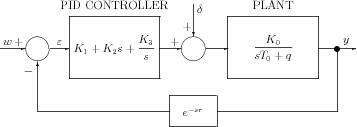]> 3.1.3 Example 3: Automatic control system with PID controller

#### 3.1.3 Example 3: Automatic control system with PID controller

The dynamics of the system depicted in Figure 3.3Figure 3.3: Automatic control system with PID controller

is governed by the equations

$\left\{\begin{array}{ccc}\hfill \varepsilon \left(t\right)& \hfill =\hfill & -y\left(t-r\right)+w\hfill \\ \hfill {T}_{0}ẏ\left(t\right)+qy\left(t\right)& \hfill =\hfill & {K}_{0}\left[\delta \left(t\right)+{K}_{1}\varepsilon \left(t\right)+{K}_{2}\stackrel{̇}{\varepsilon }\left(t\right)+{K}_{3}{\int }_{0}^{t}\varepsilon \left(\tau \right)d\tau \right]\hfill \end{array}\right\},\phantom{\rule{2em}{0ex}}t>0$

where $\delta$ denotes the Dirac pseudofunction and $w$, ${K}_{0}$, ${K}_{1}$, ${K}_{3}$, ${T}_{0}$, $r>0$ and $q$ are constants (usually $q=0$ or $q=1$).

If we assume that the system is asymptotically stable and until the appearance of the disturbance it remains in the equilibrium then for $t<0$

$\varepsilon =0,\phantom{\rule{2em}{0ex}}y=w,\phantom{\rule{2em}{0ex}}{\int }_{0}^{\infty }\varepsilon \left(t\right)dt=\frac{qw}{{K}_{0}{K}_{3}}.$

Hence denoting

$c=-\frac{{K}_{0}{K}_{2}}{{T}_{0}},\phantom{\rule{1em}{0ex}}a=-\frac{q}{{T}_{0}},\phantom{\rule{1em}{0ex}}b=-\frac{{K}_{0}{K}_{1}}{{T}_{0}},\phantom{\rule{1em}{0ex}}d=-\frac{{K}_{0}{K}_{3}}{{T}_{0}},\phantom{\rule{1em}{0ex}}{z}_{0}=\frac{{K}_{0}}{{T}_{0}},$

and introducing the system variables

${z}_{1}\left(t\right)=y\left(t\right)-w,\phantom{\rule{2em}{0ex}}{z}_{2}\left(t\right)=-d{\int }_{0}^{t}\varepsilon \left(\tau \right)d\tau +aw$

we obtain the system of equations

 $\left\{\begin{array}{cccc}\hfill \frac{d}{dt}\left[{z}_{1}\left(t\right)-c{z}_{1}\left(t-r\right)\right]& \hfill =\hfill & a{z}_{1}\left(t\right)+{z}_{2}\left(t\right)+b{z}_{1}\left(t-r\right)\hfill & \hfill \\ \hfill \frac{d}{dt}\left[{z}_{2}\left(t\right)\right]& \hfill =\hfill & d{z}_{1}\left(t-r\right)\hfill & \hfill \\ \hfill {z}_{1}\left(\theta \right)& \hfill =\hfill & 0,\hfill & -r\le \theta <0\hfill \\ \hfill {z}_{1}\left(0\right)& \hfill =\hfill & {z}_{0}\hfill & \hfill \\ \hfill {z}_{2}\left(\theta \right)& \hfill =\hfill & 0,\hfill & -r\le \theta <0\hfill \end{array}\right\}$ (3.14)

The system (3.14) has the form (3.5) with

$\begin{array}{c}n=2,\phantom{\rule{2em}{0ex}}{A}_{1}=\left[\begin{array}{cc}\hfill a\hfill & \hfill 1\hfill \\ \hfill 0\hfill & \hfill 0\hfill \end{array}\right],\phantom{\rule{2em}{0ex}}{A}_{2}=\left[\begin{array}{cc}\hfill b\hfill & \hfill 0\hfill \\ \hfill d\hfill & \hfill 0\hfill \end{array}\right],\phantom{\rule{2em}{0ex}}{A}_{0}=\left[\begin{array}{cc}\hfill c\hfill & \hfill 0\hfill \\ \hfill 0\hfill & \hfill 0\hfill \end{array}\right],\hfill \\ {v}_{0}=\left[\begin{array}{c}\hfill {z}_{0}\hfill \\ \hfill 0\hfill \end{array}\right],\phantom{\rule{2em}{0ex}}\phi \equiv 0.\hfill \end{array}$

The problem is to express explicitly the integral

 (3.15)

by the parameters $a$, $b$, $c$, $d$.